What does it do?

Formula breakdown:

=number1 + number2

What it means:

In Excel adding numbers together is really easy with the Addition Formula which uses the addition operator: +

I will show you in the steps below how you could add numbers together in your Excel worksheet.

This is the table of values that we want to perform addition on: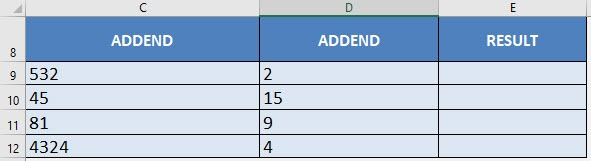I explain how you can do this below:STEP 1: We need to enter the number we want to add:

## =C9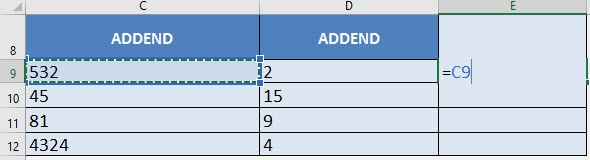STEP 2: Enter the addition operator +

## =C9 +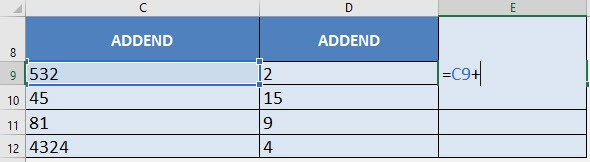STEP 3: Enter the number to add:

## =C9 + D9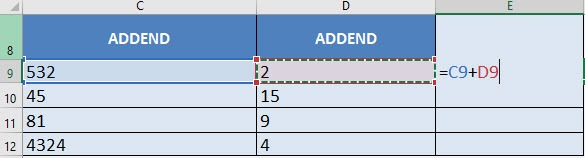Apply the same formula to the rest of the cells by dragging the lower right corner downwards.

You now have all of the addition results!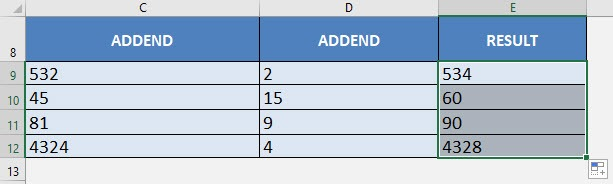How to Use the Addition Formula in Excel